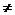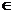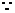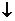2.11. Remainder Theorem:

2.11.1 Roots of an equation/Zero of a polynomial

Let us take the polynomial 4023m2n2 - 6032m2n - 8042m3 n4.

Since this polynomial contains m and n as variables we can denote the same by f(m,n).

f(m,n) is pronounced as ‘function of m and n’.

f(m,n) = 4023m2n2 - 6032m2n - 8042m3 n4

A polynomial f(x) in one variable x is an algebraic expression of the form

f(x) = anxn+ an-1xn-1+ an-2xn-2+ ………. a2x2+ a1x+ a0 = 0

where a0,a1,a2,……… an-1,an are constants and an0

a0,a1,a2,……… an-1 and an are called ‘co-efficients’ of  x0,x1,x2……. xn-1 and xn respectively. n is called the ‘degree’ of the polynomial.

Each of anxn, an-1xn-1,………. a2x2, a1x1, a0 are called the ‘terms’ of the polynomial.

Let f(x) = x5 - 9x2 + 12x - 14

If we substitute x = 0 we get f(0) =  0 -9*0 +12*0 -14 = -14

If we substitute x = 1 we get f(1) =   1-9+12-14= -10

Similarly, if we substitute x = -1 we get f(-1) =  -36

f(a) = a5 - 9a2 + 12a - 14

If for any value of a (x=a), f(x) = 0, then we say that ‘a’ is a ‘root’ of the equation f(x)=0.

If in case of polynomial f(x), if  f(a)=0, then  'a' is called 'zero' of polynomial f(x).

2.11.2 More on divisions:

We know that  like in case of numbers,  following relationship holds good in case of polynomials also.

Divisor*quotient + reminder =  dividend

Above relationship is called Euclid's Division Lemma for polynomials and we can say

f(x)= g(x)*q(x)+ r(x)  ---(1)

[ Here  the divisor g(x)divides f(x) to give the quotient q(x) and reminder  r(x). Note g(x)0 and r(x) =0 or its degree is less than degree of g(x) ]

Note   in (1) if any three polynomials are given then the fourth polynomial can be  found

If f(x), q(x) and r(x) are given then g(x)= {f(x)-r(x)}/q(x)

If f(x), g(x) and q(x) are given then r(x)= f(x)-{ g(x) *q(x)}

Can we find quotient and reminder on dividing a polynomial without the actual division?

2.11 Problem 1: Find  the quotient and reminder on dividing f(x) = x3+4x2-6x+2 by g(x)= (x-3)

Solution:

We know f(x)= g(x)*q(x)+ r(x)  and also degree of r(x) is less than that of g(x). Thus degree of g(x) has to be 0. Since degree of dividend is 3 and degree of divisor is 1, degree of quotient has to be 2(=3-1).

Hence let r(x) be = k a constant and q(x) = ax2+bx+c for some value of a, b and c.x3+4x2-6x+2 =(x-3)* (ax2+bx+c) = (ax3+bx2+cx)+(-3ax2-3bx-3c)+ k = ax3+x2(b-3a)+x(c-3b)+k-3c.a=1;4=b-3a; -6=c-3b;2=k-3c ( Equating co-efficents of respective terms)

Thus a=1; b=4+3a; c=3b-6; k=2+3c

By simplifying further we get a=1, b=7, c=15 and k= 47q(x) = ax2+bx+c= q(x) = x2+7x+15 and r(x) = 47

Verification:

Verify that x3+4x2-6x+2 = (x-3)* (x2+7x+15)+47

How to modify  a polynomial so as to be exactly divisible by another polynomial?

We know  that

f(x)= g(x)*q(x)+ r(x)

if g(x) has to divide f(x) exactly, then r(x) has to be zero

·         First divide f(x) by g(x) by any of the method( long division as learnt in the lesson 2.10 or by the method mentioned in problem 2.11.1) to get the reminder r(x).

·         Subtract this r(x) from f(x) to get a new  polynomial which is divisible exactly by g(x).

2.11 Problem 2:  What should be added to or subtracted   from x3+5x2+5x+8   to make it exactly divisible by x2+3x-2?

Solution:

When we divide x3+5x2+5x+8 by x2+3x-2 we get the reminder as  -1060

Since for exact divisibility reminder has to be zero, we need to subtract this reminder from the  dividend and hence the answer is (x3+5x2+5x+8) – (-1060)= x3+5x2+5x+1060

2.11.2 Remainder Theorem:

We notice that the method mentioned in section 2.10 to find the remainder by repeated process of divisions, is a long process and is time consuming..

Is there a simple method by which remainder can be found?

By analyzing the problems solved in Section 2.10 we can observe a few things.

In case of the Problem 2.10.3.1  where we divided (x3 -6x+7)  by  (x+1)

let us call dividend as f(a) {pronounced as function of a}then

f(a) = a3-6a +7

Let us find the values of f(a) for various values of a (say 1, 2,0,-1,-2)

Then we have

f(1) =  2, f(0) =7, f(-1) = 12, f(-2) = 11.

What do we observe? We notice f(-1)=12 is the remainder.

Let us take the problem of  dividing of  (x4-2x3+x-7)  by  (x+2)

let us call dividend as f(x) (function of x) then

f(x) = x4-2x3+x-7

Let us find the value of f(x) for various vales of x (say 1, 2, 0,-1,-2)

Then we have

f(1) =  -6, f(2)  =-5,  f(0)  =-7, f(-1) =-7,  f(-2)=23  is the remainder

Let us do some tabulation of remainders for various values of dividends and divisors

 Dividend- f(x) Divisor g(x) Quotient q(x) Remainder r(x) Remainder=  value of function f(k) x3-6x +7 x+1 x2-x-5 12 f(-1) x4-2x3+x-7 x+2 x3-4x2+8x-15 23 f(-2) x+1 x+1 1 0 f(-1) x-1 x-1 1 0 f(1) x+a x+a 1 0 f(-a) x-a x-a 1 0 f(a) x2+4x+4 x+2 x+2 0 f(-2)

We conclude that if a polynomial f(x) is divided by a monomial of type (x+a) then the remainder is equal to f(-a)

This is called Remainder Theorem:

If a Polynomial f(x), over the set of real numbers R  is divided by (x+a)  then the remainder is f(-a).

Note: “over the set of real numbers” means that x can take the value of any real number (xreal number)

Proof of Remainder theorem:

Remainder theorem states that if a Polynomial f(x), is divided by (x+a) then the remainder is f(-a).

Let q(x) and r(x) be the quotient and the remainder when we divide f(x) by x+a.

Proof:

We have Dividend = Quotient*Divisor + Remainderf(x) = q(x)*(x+a) + r(x)

Note that the degree of the divisor (=(x+a)) is 1.

Also note that the degree of remainder (= r(x)) has to be less than the degree of divisor.

Hence, the degree of remainder = 0 which means that r(x) does not contain the term x and so it has to be a constant (say ‘r’).f(x) = q(x)*(x+a)+r

Since the above equation is true for any value of x, it has to be true for x = -a also.f(-a) = q(-a)*(-a+a)+r = q(-a)*0+r = r

This proves the theorem.

Note: If a Polynomial f(x), is divided by (ax+b) then the remainder is f(-b/a) [ax+b = (x+b/a) ]

Factor Theorem:

If  f(-a) is equal to 0  then (x+a) is a factor of the polynomial f(x)

Proof:

Suppose f(-a) = 0

By remainder theorem, on dividing f(x) by (x+a), the remainder is f(-a). So from the fact that f(-a)=0, it follows that the remainder on dividing f(x) by x+a is 0. This means that x+a divides f(x) exactly. That is x+a is a factor of f(x).

2.11 Problem 3: Find remainder of   x3+2x2-x+6 when divided by x-3

Solution:

Here f(x) = x3+2x2-x+6 and divisor is x-3

As per the remainder theorem, if divisor is of the form x+a then the remainder is f(-a).

Since divisor is of the form x+a , f(-(-3) = f(3) is the remainder

Substituting 3 in f(x), we get

f(3) = 27+ 18-3+6 = 48 is the remainder

2.11 Problem 4: Find value of a if x+2 is a factor of 4x4+2x3-3x2+8x+5a

Solution:

Since x+2 is a factor of f(x), remainder has to be 0.

Thus by Remainder theorem f(-2) =0

f(-2) = 4*16+2*(-8)-3*4 -16+5a = 64-16-12-16+5a = 20 +5a

Since f(-2) =0 we have 20+5a = 0 i.e. 5a = -20 i.e. a= -4

Verification:

Since a= -4, substitute -4 for a in f(x)

We get f(x) = 4x4+2x3-3x2+8x-20

f(-2)  = 4*16+2(-8)-3*4 -16 -20 = 64-16-12-16-20 = 0

This proves that x+2 is a factor of 4x4+2x3-3x2+8x-20

2.11 Problem 5: Check whether 7+3x is a factor of 3x3+7x

Solution:

Let f(x) = 3x3+7x

If 7+3x has to be a factor of f(x), then 3*(7/3+x) has to be necessarily a factor of f(x) (If y is a factor of f(x) then ny/n has to be a factor of f(x) as y*f(x) = (ny/n)*f(x) when n is any non zero number)f(-7/3) = 3(-7/3)3 +7(-7/3) =  -343/9 -49/30

Thus 7+3x is not a factor of the given polynomial.

Note: 3x3+7x = x(3x2+7) which clearly indicates that 7+3x is not a factor.

2.11 Problem 6: Check if 0, 1, 2 are roots of the equation x2-2x=0

Solution:

Let f(x) = x2-2x

We note that f(0) = 02-2*0 = 0,

f(1) = 12-2 = -1

f(2) = 22-2*2 = 0

Thus 0 and 2 are the roots of the given polynomial but 1 is not.

2.11  Problem 7: If f(x) = x2+5x+p and q(x) = x2+3x+q have a common factor then

(i) Find the common factor

(ii) Show that (p-q)2= 2(3p-5q)

Solution:

Since degree of f(x) is 2 and it has a common factor, the degree of the factor has to be one.

Let it be x-kf(k) = k2+5k+p = 0

Since x-k is also a factor of q(x)q(k) = k2+3k+q = 0k2+5k+p = k2+3k+q: On simplificationk = (1/2)(q-p)

Hence the common factor = x-k = x - (1/2)(q-p)

= x + (1/2)(p-q)

By substituting the value of k in f(x) we get

((q-p)/2)2+5(q-p)/2+p = 0

i.e. (p-q)2/4+5(q-p)/2+p = 0

i.e. (p-q)2+10(q-p)+4p = 0

i.e. (p-q)2 = 10p-10q-4p

= 6p-10q

= 2(3p-5q)

2.11 Problem 8: Factorise 6x2-11x+3 given that  f(1/3) and f(3/2)=0

Solution:

Let f(x) = 6x2-11x+3

It is  given that  (x-1/3) and (x-3/2) are factors of f(x)

(x-1/3)*(x-3/2)

= x2 - (1/3)x (3/2)x + 3/6

= x2 - (11/6)x +3/6

= (6x2-11x+3)/6.

Multiply both sides by 6 we getf(x) = 6x2-11x+3 = 6(x-1/3)*(x-3/2)

2.11 Problem 9: If (x+1) is a factor of x3 +2x2 - 5x – 6, find its other factors.

Hint:

Let f(x) = x3 + 2x2- 5x - 6

We also note that f(-1) = -1+2+5-6 = 0 thus clearly (x+1) is a factor of f(x).

Using the division method learnt in section 2.10, we find that

f(x) = (x+1)(x2+x-6)

By factorizing,

(x2+x-6)

= (x2+3x-2x-6)

= x(x+3)-2(x+3)

= (x+3)(x-2)

Hence

f(x) = (x+1)(x-2)(x+3)

2.11 Problem 10: If a quadratic polynomial, when divided by (x-1), (x+1) and (x-2) leaves the remainders 2, 4 and 4 respectively, find the quadratic equation.

Solution:

Let the quadratic polynomial be f(x) = ax2+bx+c

From the given data f(1)=2, f(-1)=4 and f(2)=4

But f(1) = a+b+c, f(-1) = a–b+c and f(2) = 4a+2b+cWe have

a+b+c = 2

a-b+c = 4

4a+2b+c = 4

When we solve these three simultaneous equations as explained in 2.14.3 we get

a=1, b=-1 and c=2

Hence the quadratic equation is x2-x+2.

2.11 Problem 11: Given that px2+qx+6 leaves a remainder of 1 when divided by 2x+1 and 2qx2+6x+p leaves a remainder of 2 when divided by 3x-1, find p and q.

Solution:

Let  f(x) = px2+qx+6, g(x) = 2qx2+6x+p

When f(x) is divided by 2x+1, the remainder is 1. This implies that f(-1/2) = 1p/4 –q/2+6 = 1

i.e. p-2q = -20 (On simplification)    ---à(1)

When g(x) is divided by 3x-1, the remainder is 2. This implies that g(1/3) = 22q/9 + 6/3 +p = 2

i.e. 9p+2q = 0 (On simplification)      ---à(2)

On solving (1) and (2) we get

p = -2 and q = 9

Synthetic method (Horner’s method) of division when the divisor is of the form x-a.

Using this method we can find quotient and reminder easily compared to long division method.

We shall describe this method by taking the problem which was worked out earlier (2.10.3 Problem 2).

Divide x5 -9x2 +12x-14 by x -3

Solution:

Write the dividend in its standard form as: 1x5 + 0x4 + 0x3 - 9x2 + 12x - 14.

Here the constant term in the divisor is -3

1.      First, write the –negative of constant term in the divisor (3 in this case) in the first column of the first row. In the next columns of the first row write the co-efficients of the dividend (1, 0, 0, -9, 12, -14)

2.      Write the co-efficient of the first term of the divisor (in this case 1) in the corresponding column of the third row down below (in 2nd column).

3.      starting from this column in the third row, write the product of divisor (in this case 3) and the number in this column (in this case 1) in the next column of 2nd row (in this case 3*1=3 in the 3rd column).

4.      Add these numbers in the 1st and 2nd row (in this case 0+3=3) into the corresponding column in the third row.

5.      Repeat this process till the result in the last column in the third row is got.

6.      The value in the last column of the third row gives the reminder.

7.      Other values in the third row  represent  the co-efficient of the quotient.

 Divisor Dividend portion(Columns from 2 onwards) 3 1 0 0 -9 12 -14 First Row3(=3*1) 9(= 3*3) 27(= 3*9) 54(= 3*18) 198(= 3*66) Second row 1 3=(0+3) 9(= 0+9) 18(=-9+27) 66(=12+54) 184(=-14+198) Third row

You will observe that the quotient is 1x4+3x3+9x2+18x  and reminder is 184, which is same as what we got while solving problem (2.10.3 Problem 2)

2.11 Summary of learning

 No Points studied 1 If a polynomial f(x) is divided by (x+a) then remainder = f(-a) for any real value of x 2 If a polynomial f(x) is divided by the divisor of the form x+a and the remainder is zero, then  x+a is a factor of f(x) 3 If f(-a) is equal to 0  then (x+a) is a factor of the polynomial f(x)